• matlab开发-形结构类。实现通用树数据结构的每个值类。图像处理与计算机视觉
• matlab开发-单元格结构树和结构排序。从单元格或结构为单元格数组、结构和数据生成树。
• 数据结构 该软件包包含以下常见数据结构的基于MATALB类的实现： 1）数组 2）二进制搜索 3）哈希表 4）堆 5）列表 6）队列 7）红黑 8）堆栈 编写代码的方式很容易将其翻译成其他语言。 完整资料领取：...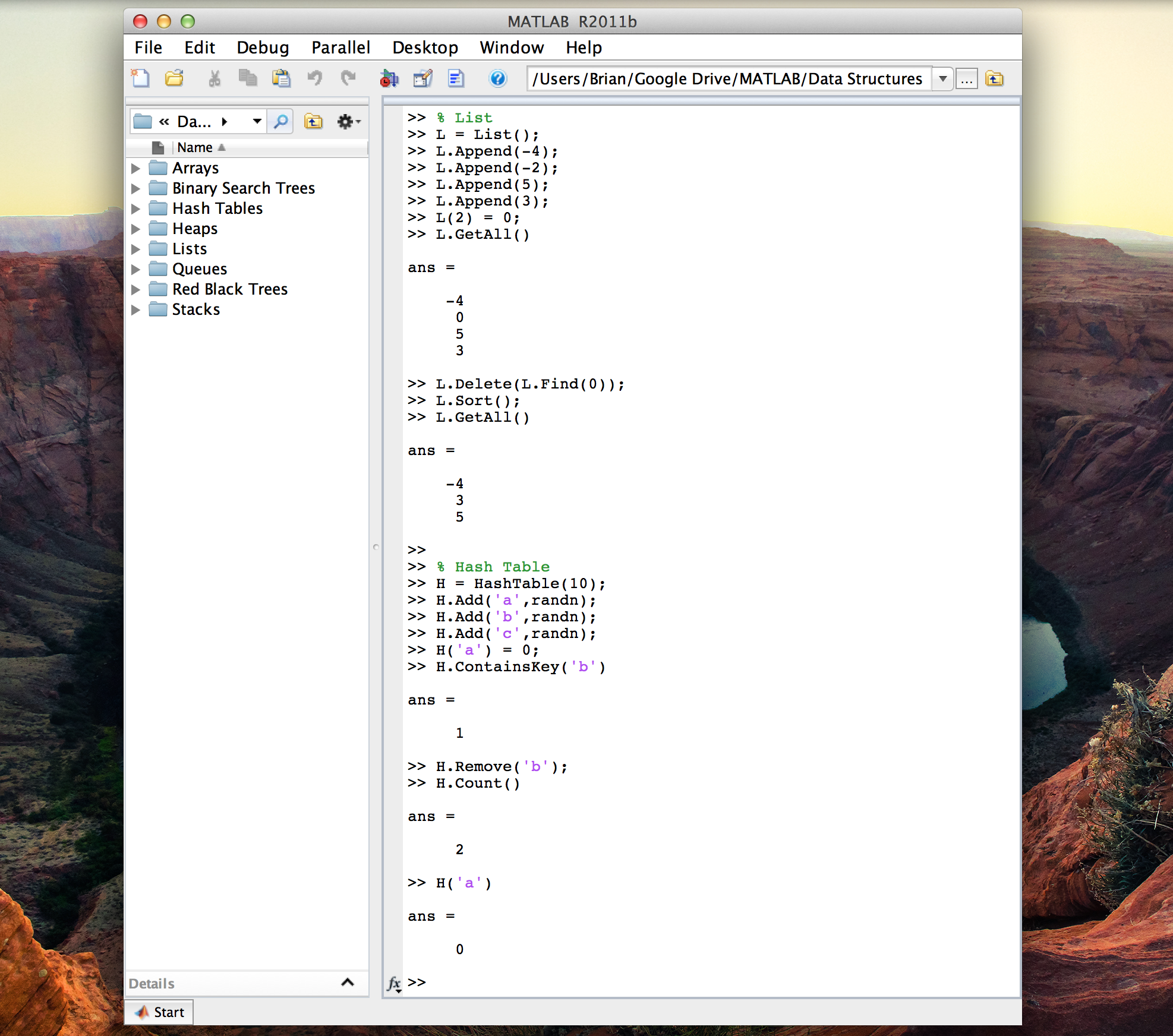数据结构

该软件包包含以下常见数据结构的基于MATALB类的实现：

1）数组
2）二进制搜索树
3）哈希表
4）堆
5）列表
6）队列
7）红黑树
8）堆栈

编写代码的方式很容易将其翻译成其他语言。

完整资料领取：https://ai.52learn.online/9669

展开全文• 本博客的数据和要求是我的数据挖掘课老师提供的，代码是个人原创的。联系方式vx:qczsbwjzjn，如果想要数据可以找我。另外安利一下Matlab用于查询函数用法的官网https://ww2.mathworks.cn/help/matlab/ 一、要求 ...
本博客的数据和要求是我的数据挖掘课老师提供的，代码是个人原创的。联系方式vx:qczsbwjzjn，如果想要数据可以找我。另外安利一下Matlab用于查询函数用法的官网https://ww2.mathworks.cn/help/matlab/

一、要求

给定2013,2014,2015,2016,2017的福彩双色球数据，共767期数据
(1) 使用一种编程语言实现FP-tree的建树算法，并应用于此数据构建FP-tree；
(2) 统计所建FP-tree中的节点数目，包括根节点；
(3) 通过使用一个算法给出从根到叶子的节点数最多一条路径，并显示其中除根以外每个节点上的支持度计数;
(4) 给出关于根到叶子的节点一条路径，且该路径上所有节点的支持度计数之和最大，显示每个节点上的支持度计数及总和。

二、数据格式

示例：

期号       红球1    红球2    红球3    红球4    红球5    红球6

2013001    6          8           14         15          24         25
2013002    1         16          18         22          28         30
2013003    22       23          26         27          28         33
2013004    6         10          16         20          27         32

三、数据结构

用一个1*n的cell来存放一个节点的信息

cell{1,1}储存当前节点所对应的球编号，cell{1,2}储存当前节点的支持度计数，cell{1,m}(2<m<=n)指向该节点下的子节点

如图所示为根节点的节点信息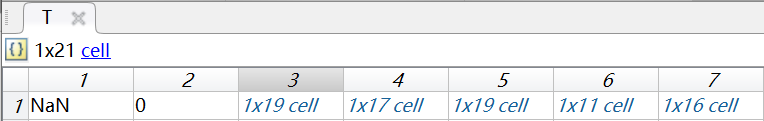四、Matlab代码

main.m 主函数

clear
%% 主程序
%% 读取数据
ball=importdata('作业01-双色球数据ssq2013_2017.txt');
ball=ball.data(:,2:end);%提取有效数据 得到767*6的矩阵
%%
f=tabulate(ball(:));
f=sortrows(f,-2);%按支持度降序排列
%项头表，第一列是球编号，第二列是支持度，第三列是占总体的比例
%% 设置支持度阈值
max=f(1,2);%支持度最大值
threshold=max*0.8;%支持度阈值
d=f(f(:,2)<=threshold,1);%提取出支持度低于阈值的球编号至d
f(f(:,2)<=threshold,:)=[];%删去支持度低于阈值的球编号
order=f(:,1);%排列顺序
for i=1:length(ball)
[~,index]=ismember(ball(i,:),order);
temp=[ball(i,:);index];
temp(:,temp(2,:)==0)=NaN;
temp=sortrows(temp',2)';
ball(i,:)=temp(1,:);
end
%% 初始化根节点
T{1,1}=NaN;
T{1,2}=0;
%% 建立FP树
for i=1:length(ball)
temp=ball(i,:);%temp为当第i行数据
temp=temp(~isnan(temp));%删去NaN
if ~isempty(temp)%temp不空，向T中插入temp
T=insertChild(T,temp,1);
end
end
%% 统计节点数目，包括根节点
Node_number=nodenumber(T);

%% 统计节点最多的路径，并记录其每个节点的支持度
% 第一行为球的编号 第二行为编号对应的支持度
% 编号NaN表示根节点
Node_max=nodemax(T);
%% 节点支持度之和最大的路径
Node_support=supportmax(T);

insertChild.m 【递归】插入子树

function cell=insertChild(cell,num,k)
loc=isChild(cell,num(k));
if loc~=0%该元素是子树
cell{1,loc}{1,2}=cell{1,loc}{1,2}+1;
if k~=length(num)
cell{1,loc}=insertChild(cell{1,loc},num,k+1);
end
else %该元素非子树
if k==length(num)
child{1,1}=num(k);
child{1,2}=1;
cell{1,length(cell)+1}=child;
else
len=length(cell);
cell{1,len+1}{1,1}=num(k);
cell{1,len+1}{1,2}=1;
cell{1,len+1}=insertChild(cell{1,len+1},num,k+1);
end
end
end

isChild.m 判断该节点是否是子树，并返回位置

function loc=isChild(cell,num)
%loc表示该元素在cell中的位置
loc=0;
if length(cell)>2
for i=3:length(cell)
if isempty(cell{1,i})
break
elseif cell{1,i}{1,1}==num
loc=i;
break;
end
end
end
end

nodenumber.m文件 【递归】寻找当前树的叶子节点个数

function y=nodenumber(T)
len=length(T);
if len==2%当前节点为叶子节点
y=1;
else
y=1;
for i=3:len
y=y+nodenumber(T{1,i});
end
end
end

nodemax.m函数  【递归】寻找节点数最多的路径

function y=nodemax(T)
len=length(T);
if len==2%当前节点为叶子节点
y=[T{1,1};T{1,2}];
else
k=[T{1,1};T{1,2}];
max=1;
for i=3:len
temp=[k nodemax(T{1,i})];
if length(temp)>max
max=length(temp);
y=temp;
end
end
end
end

supportmax.m函数 【递归】寻找支持度最大的路径

function y=supportmax(T)
len=length(T);
if len==2%当前节点为叶子节点
y=[T{1,1};T{1,2}];
else
k=[T{1,1};T{1,2}];
max=0;
for i=3:len
temp=[k supportmax(T{1,i})];
if sum(temp(2,:))>max
max=sum(temp(2,:));
y=temp;
end
end
end
end

demo 简短的方便查看结果的样例代码

ball=[2 7 5;
3 6 1;
7 5 3;
6 2 3;
7 4 5];
threshold=2;%支持度阈值
f=tabulate(ball(:));        %编号  支持度
f=sortrows(f,-2);           %  3      3
d=f(f(:,2)<threshold,1);    %  5      3
f(f(:,2)<threshold,:)=[];   %  7      3
order=f(:,1);               %  2      2
%  6      2
%  1      1    （低于阈值）
%  4      1    （低于阈值）
%%
for i=1:length(ball)                    % 去除支持度低于2的元素后，按支持度对每行ball重排
[~,index]=ismember(ball(i,:),order);% [5,7,2;
temp=[ball(i,:);index];             %  3,6,NaN;
temp(:,temp(2,:)==0)=NaN;           %  3,5,7;
temp=sortrows(temp',2)';            %  3,2,6;
ball(i,:)=temp(1,:);                %  5,7,NaN]
end
%% 初始化根节点
T{1,1}=NaN;
T{1,2}=0;
%% 建立FP树                                   %%      所得树
for i=1:length(ball)                              %         NaN
temp=ball(i,:);%temp为当第i行数据              %        /  \
temp=temp(~isnan(temp));%删去NaN              %       5    3
if ~isempty(temp)%temp不空，向T中插入temp      %       |  / | \
T=insertChild(T,temp,1);                 %       7 6  5  2
end                                          %       |    |  |
end                                              %       2    7  6
%% 结果输出
%统计节点数目，包括根节点
Node_number=nodenumber(T);
% 统计节点最多的路径，并记录其每个节点的支持度
% 第一行为球的编号 第二行为编号对应的支持度
% 编号NaN表示根节点
Node_max=nodemax(T);
% 节点支持度之和最大的路径
Node_support=supportmax(T);


展开全文• 决策分类是数据挖掘中分类分析的一种算法。顾名思义，决策是基于“结构来进行决策的，是人类在面临决策问题时一种很自然的处理机制。例如下图一个简单的判别买不买电脑的决策：下图是一个测试数据集，我们...
决策树分类是数据挖掘中分类分析的一种算法。顾名思义，决策树是基于“树”结构来进行决策的，是人类在面临决策问题时一种很自然的处理机制。例如下图一个简单的判别买不买电脑的决策树：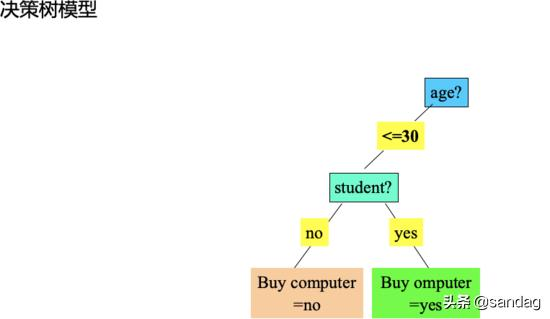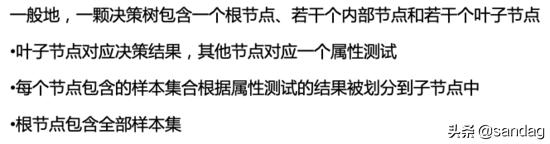下图是一个测试数据集，我们以此数据集为例，来看下如何生成一棵决策树。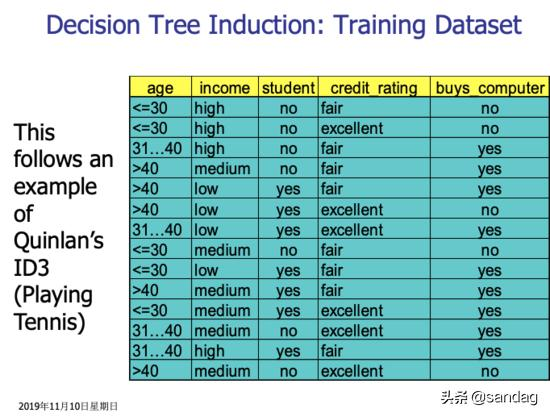决策树分类的主要任务是要确定各个类别的决策区域，或者说，确定不同类别之间的边界。在决策树分类模型中，不同类别之间的边界通过一个树状结构来表示。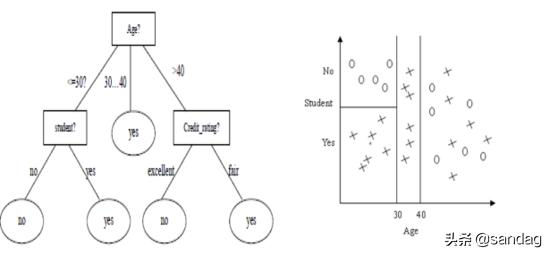通过以上分析，我们可以得出以下几点：最大高度=决策属性的个数树 越矮越好要把 重要的、好的 属性放在树根因此，决策树建树算法就是： 选择树根的过程第一步，选择属性作为树根比较流行的属性选择方法： 信息增益信息增益最大的属性被认为是最好的树根在选择属性之前，我们先来了解一个概念： 熵 什么是熵？什么是信息？如何度量他们？熵 用来表示不确定性的大小信息 用来消除不确定性实际上，给定训练集S，信息增益代表的是在不考虑任何输入变量的情况下确定S中任一样本所属类别需要的信息(以消除不确定性)与考虑了某一输入变量X后确定S中任一样本所属类别需要的信息之间的差。差越大，说明引入输入变量X后，消除的不确定性，该变量对分类所起的作用就越大，因此被称为是好的分裂变量。换句话说，要确定S中任一样本所属类别，我们希望所需要的信息越少越好，而引入输入变量X能够减少分类所需要的信息，因此说输入变量X为分类这个数据挖掘任务带来了信息增益。信息增益越大，说明输入变量X越重要，因此应该被认为是好的分裂变量而优先选择。因此，计算信息增益的总的思路是：1) 首先计算不考虑任何输入变量的情况下要确定S中任一样本所属类别需要的熵Entropy(S)；2) 计算引入每个输入变量X后要确定S中任一样本所属类别需要的熵Entropy (X,S);3) 计算二者的差，Entropy (S) - Entropy (X, S)，此即为变量X所能带来的信息(增益)，记为Gain(X,S)。结合上面对于熵的解释的文章里，我们能得出求熵的公式：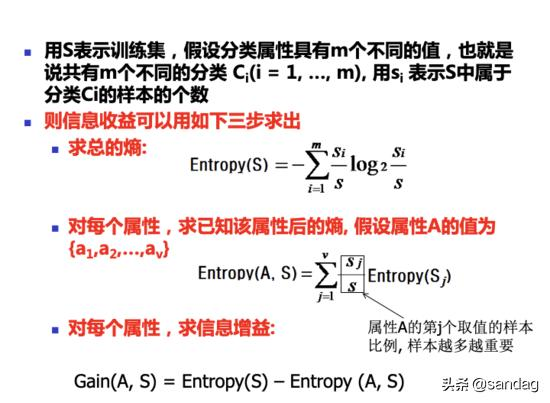下图很形象的解释了熵代表的含义。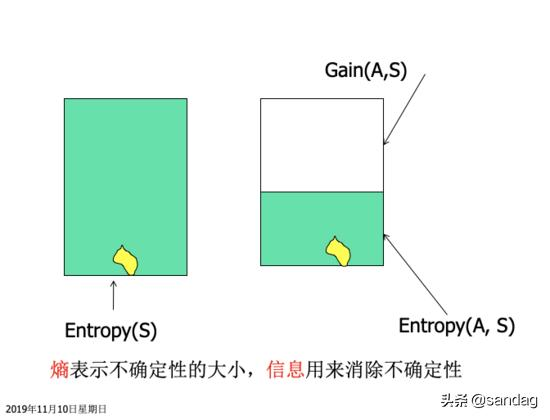我们还以上面的一组数据来分析，信息增益具体应该怎么算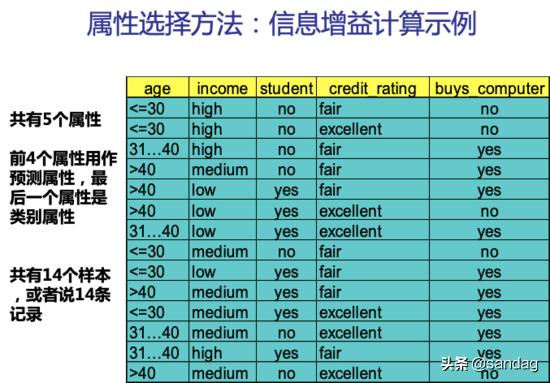根据上面的讨论，我们先用公式计算不考虑任何输入属性时，要确定训练集S中任一样本所属类别需要的熵。此例子中，目标属性即buys_computer，有2个不同的取值，yes和no，因此有2个不同的类别(m=2)。设P对应buys_computer=yes的情况，N对应buys_computer=no的情况，则P有9个样本，N有5个样本。所以，总的熵就是：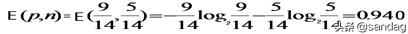即，E(p,n) = E(9,5) = 0.940然后我们来求属性age的熵，age有三个属性，样本个数分别为5，4，5，所以属性age的熵就是：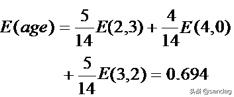最后，我们可以求出属性age的信息增益为：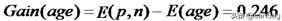同样的，我们可以分别求出income，student和credit_rating的信息增益finally，我们可以得出属性age的信息增益最大，所以，应该用属性age作为树根。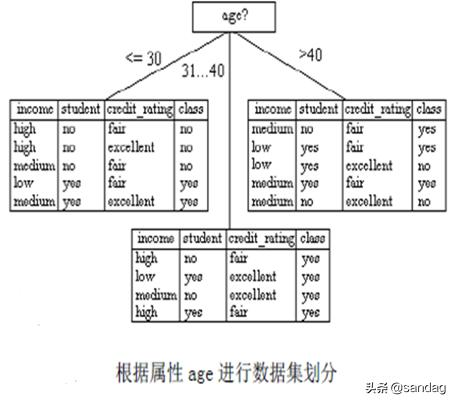确定好树根之后，下一步我们还要按照刚才的步骤来确定下一个节点的左右子树分别用哪个属性作为树根，直到最后得出完整的决策树。虽然决策树分类算法可以快速的预测分类，但是也会有过度拟合(Overfitting)的问题。有些生成的决策树完全服从于训练集，太循规蹈矩，以至于生成了太多的分支，某些分支可能是一些特殊情况，出现的次数很少，不具有代表性，更有甚者仅在训练集中出现，导致模型的准确性很低。通常采用剪枝的方式来克服 overfitting，剪枝有两种方法：先剪:构造树的过程中进行修剪。不符合条件的分支则不建。后剪: 整个树生成之后进行修剪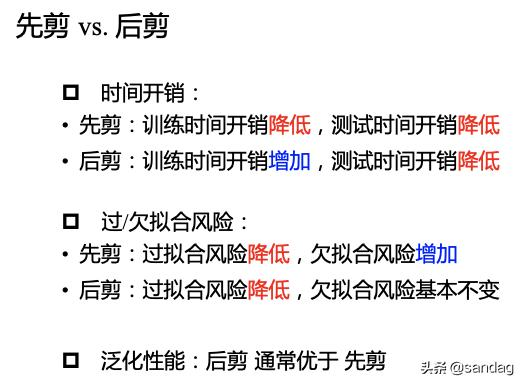展开全文• 模糊模式（FPT） 是一种机器学习的分类方法，类似于二元决策（binary descision diagram,BDD）。其内部节点使用广义（模糊）逻辑和算数运算符标记，叶节点与给定输入...BDD是用来表达布尔函数的一种数据结构。是一
一、简介
模糊模式树（FPT） 是一种机器学习的分类方法，类似于二元决策树（binary descision diagram,BDD）。其内部节点使用广义（模糊）逻辑和算数运算符标记，叶节点与给定输入属性集合上的（一元）模糊谓词相关联
模糊模式树（FPT）
在预测性能方面与其他机器学习竞争的方法相比是有竞争力的，由于物联网节点处理资源有限，他倾向于生成在解释方面非常有吸引力的紧凑模型。
其缺点也很明显:其计算复杂度高，运行时间可能变得令人无法接受。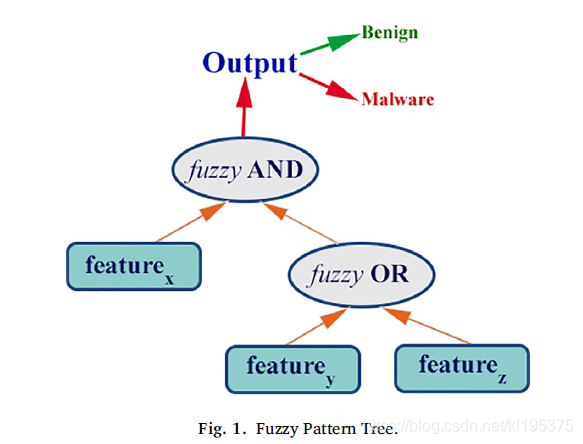二分决策树（BDD）
BDD是用来表达布尔函数的一种数据结构。是一种基于shannon分解的有向无环图。
设f为一组布尔变量集合的布尔函数（boolean function），且x为集合X中的变量，则shannon分解及其if-then-else(ite)形式为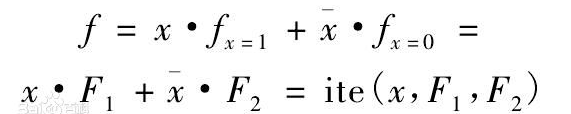式中x和 x-bar 分别代表事件发生，事件不发生；fx=1（即F1）和fx=0（即F2）
分别代表了事件发生与事件不发生的布尔函数
二叉树表示为以下：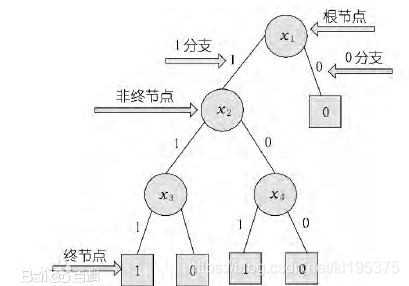布尔函数（boolean function）
布尔函数基于对布尔输入，通过某种逻辑计算，输出布尔值。
F（b1,b2,b3,…,bn）
带有n个两元素布尔代数{0,1}的布尔变量bi，F的值也是在{0,1}中
一般定义域上值在{0,1}中的函数也被称为布尔值函数，所以布尔函数是特殊情况
二、运行结果
%%----- Main Program that should be run inorder to execute the code -----%%

% load the data file
load('earfeature15window700image.mat');

% Change following variable in case of number of user changes
noOfUsers = 100;

% Change following two variable in case you need to change ration of test
% and train case or when the number of data value for same user changes(right now its 7)
trainCasePerUser = 4;
testCasePerUser = 3;

if(testCasePerUser < 2)
disp('testCasePerUser should be greater then 1')

else
totalCasePerUser = trainCasePerUser + testCasePerUser;
% 3D matrix where 1st dimention is related the user, 2nd dimention related
% to datavalues per user and 3rd dimention are the feature values for datapoints
test = zeros([noOfUsers testCasePerUser 35]);
train = zeros([noOfUsers trainCasePerUser 35]);
%% this loop devides the data into testcases and trainingcase randomly
for i=1:noOfUsers

% matrix p is the random permutation of interger 1 to total number of datavalues per user
p = randperm(totalCasePerUser,totalCasePerUser);

% below two lines divide the data into tests and training data
test(i,:,:) = T_S1(:,(i-1)*totalCasePerUser + p(1:testCasePerUser))';
train(i,:,:) = T_S1(:,(i-1)*totalCasePerUser + p(testCasePerUser+1:totalCasePerUser))';
end
%% Following piece of code find creates the matching score matrix with 2 columns
% 1st column has the value of matching score and 2nd column has value 1 if
% its genuine and 0 if imposter
trainScore = zeros([noOfUsers*noOfUsers 2]);
testScore = zeros([noOfUsers*noOfUsers*(testCasePerUser-1) 2]);
% loop that creates the matrix of training sample.
for i=1:noOfUsers
for j=1:noOfUsers
score = zeros([trainCasePerUser 1]);
for k = 1:trainCasePerUser
score(k) = norm(squeeze((test(i,1,:) - train(j,k,:))),2);
end

% take the min of distance to get the matching score
trainScore((i-1)*noOfUsers + j,1) = min(score);

%  if the users are same then its genuine class else imposter
if(i==j)
trainScore((i-1)*noOfUsers + j,2) = 1;
else
trainScore((i-1)*noOfUsers + j,2) = 0;
end
end
end
% loop that creates the matrix of testing sample
for z=0:testCasePerUser-2
for i=1:noOfUsers
for j=1:noOfUsers
score = zeros([trainCasePerUser 1]);
for k = 1:trainCasePerUser
score(k) = norm(squeeze((test(i,z+2,:) - train(j,k,:))),2);
end
testScore(z*noOfUsers*noOfUsers + (i-1)*noOfUsers + j,1) = min(score);
if(i==j)
testScore(z*noOfUsers*noOfUsers + (i-1)*noOfUsers + j,2) = 1;
else
testScore(z*noOfUsers*noOfUsers + (i-1)*noOfUsers + j,2) = 0;
end
end
end
end
% find the geninue class datapoint and put them in Gtrain Matrix
Gtrain = trainScore(find(trainScore(:,2) == 1), :);
% find the imposter class datapoint and put them in Itrain Matrix
Itrain = trainScore(find(trainScore(:,2) == 0), :);
% Create the final training matrix with  score value in 1st column, membership
% value in 2nd column and class in 3rd column for creating dicision tree.
% change the membership function 2nd  column in case you want to use
% different membership funtion
Mtrain = [Gtrain(:,1) GaussianMembership(Gtrain(:,1)) Gtrain(:,2); Itrain(:,1) TrapezoidalMembership(Itrain(:,1)) Itrain(:,2)];
%Mtrain = [Gtrain(:,1) TrapezoidalMembership(Gtrain(:,1)) Gtrain(:,2); Itrain(:,1) TrapezoidalMembership(Itrain(:,1)) Itrain(:,2)];
%% This part of code deals with growing the decision tree
% sort the training matrix according the matching score value.
[a b] = sort(Mtrain(:,1));
clear a;
data = Mtrain(b,:);
% grow the tree using sorted training matrix
tree = growTree( data );
%% This part of code deals with the predicting the class of testing score and
% calculation of FRR and FAR
testingSize = noOfUsers*noOfUsers*(testCasePerUser-1);
geniuneTestingSize = noOfUsers*(testCasePerUser-1);
trainingSize = noOfUsers*noOfUsers;
geniuneTrainingSize = noOfUsers;
pre = zeros([testingSize 2]);
% loop that predicts the values of all testing data
for i=1:testingSize
pre(i,1) = predict(tree, testScore(i,:));
pre(i,2) = testScore(i,2);
end
% calculation of FAR and FRR
FA = pre(find(pre(:,1) == 1 & pre(:,2) == 0),:); % False Acceptance
FR = pre(find(pre(:,1) == 0 & pre(:,2) == 1),:); % False Rejection
FAR = size(FA,1)/(testingSize-geniuneTestingSize); % False Acceptance Rate
FRR = size(FR,1)/geniuneTestingSize; % False Rejection Rate
%% histgram of normalized frequencies of matching score of Geniune and Imposter matching score
figure;
[m,yout] = hist(Itrain(:,1), 20);
m = m/(size(Itrain,1));
[n,xout] = hist(Gtrain(:,1), 20);
n = n/size(Gtrain,1);
bar(yout,m);
hold on;
bar(xout,n, 'r');
legend('Imposter Scores','Geniune Scores');
hold off;
end

三、备注
完整代码或者代写添加QQ2449341593
往期回顾>>>>>>
【数学建模】基于matlab SEIRS传染病模型【含Matlab源码 033期】
【数学建模】基于matlab元胞自动机的人口疏散仿真【含Matlab源码 035期】
【数学建模】基于matlab粒子群优化ELM网络预测【含Matlab源码 036期】
【数学建模】基于matlab时变参数随机波动率向量自回归模型（TVP-VAR）【含Matlab源码 037期】


展开全文• 我比较推荐清华大学邓俊辉老师的数据结构，浙江大学的数据结构也很好。其实看看离散数学也行。内存中数据的存储是没有链表、、图的。这些内容就是抽象出来的。同理，我们也不知道c语言里的数组、结构体是如何...
• 算法介绍回归决策主要指CART(classification and regression tree)算法，内部结点特征的取值为“是”和“否”，为二叉树结构。所谓回归，就是根据特征向量来决定对应的输出值。回归就是将特征空间划分成若干单元...
• 模糊模式（FPT）是一种机器学习的分类方法，类似于二元决策（binary descision diagram,BDD）。其内部节点使用广义（模糊）逻辑和算数运算符标记，叶节点与给定输入属性...BDD是用来表达布尔函数的一种数据结构。.
• 笔记01【第1、2章】【概述、软件介绍】笔记02【第3章】【数据处理方法】笔记03【第4章】【规划模型】笔记04【第5章】【图与网络模型】各个章节---作业题解析目 录5.1 图的基本概念与数据结构5.1.1 图的基本概念5.1.2...
• ## 决策树 for Matlab

千次阅读 2018-03-20 11:43:24
决策 in Matlab决策是一种常见的分类决策结构。在获取足够的数据条件下，根据筛选特征的先验条件分布，生成一种能够帮助决策的树结构。生成决策的过程，可以由Matlab帮助生成。假设输入的样本含有N维特征和一...
• 1，用过matlab生物工具箱的都知道，里面有发育函数，就给定特殊结构数据可以算出发育（具体参见帮助文档Bird Flu的demo）,在实例中是用view（tree），去打开该发育。我之前以为这种只能用这种特定的工具...
• 基于MATLAB的简单语音系统处理摘要：语音数据的有效编码可以提高通信系统的有效性，大大减少存储设备的容量。子带编码是一种常用语音编码技术，子带编码中的子带分解和合成是子带编码中的重要组成部分。本文提出了...plot filter function signal
• MATLAB-绘制网络拓扑结构星形拓扑结构定义.m文件形拓扑结构定义.m文件 参考文档连接： 1.拓扑结构的介绍 星形拓扑结构 定义 星型拓扑结构是一个中心，多个分节点。它结构简单，连接方便，管理和维护都相对容易，...
• 决策是一种常见的机器学习方法，以二分任务为例，我们希望从给定训练数据集学得一个模型用以对新示例进行分类，顾名思义，这个分类的任务是基于结构来决策的，这恰是人类在面临决策问题时一种很自然的处理机制...
• 用于建立kd树数据结构，对于做图像处理的人应该很有用kdtree
• 学过数据结构的都知道“最小生成” (Minimum Spanning Tree)，可以使用Prim和Kruskal算法找到完全图的最小生成。但在有些时候还需要找最大带权生成 (Maximum Weighted Spanning Tree)，例如在西瓜书（即 ...
• ## 决策树---MATLAB程序

千次阅读 2019-04-07 20:32:35
决策模型呈结构，在分类问题中，表示基于特征对实例进行分类的过程。它可以认为是if-then规则的集合，也可以认为是定义在特征空间与类空间上的条件概率分布。其主要优点是模型具有可读性，分类速度快。学习时...
• matlab开发-字段名称的指定字段结构的提取值。用于搜索和收集具有特定字段名的树数据类型结构中的值的有用工具
• ## 决策树的实现原理与matlab代码

万次阅读 多人点赞 2016-10-23 10:55:09
很久不写博客了，感觉很长一段时间只是一味的看书，疏不知一味地看书、写代码会导致自己的思考以及总结能力变得衰弱。...决策是一种对实例分类的结构中包含叶子节点与内部节点。内部节点主要是数据中的某一特
• k-d（k-dimensional的简称），是一种分割k维数据空间的数据结构。主要应用于多维空间关键数据的搜索（如：范围搜索和最近邻搜索）。  这里用的是在VLFeat 实现的kd，其是一个开源的计算机视觉库，实
• 今天是算法和数据结构专题20篇文章，我们继续最小生成算法，来把它说完。在上一篇文章当中，我们主要学习了最小生成的Kruskal算法。今天我们来学习一下Prim算法，来从另一个角度来理解一下这个问题。从边到点...
• 第一次学数据结构是在大二的时候了，那时候一口气学到了图，计算最小生成什么的，不过那会都是用的c语言和matlab，也没保存下来，这会也忘记很多了，期间还刷了不少leetcode的题目但也很少记下来==，所以这次就...
• maketree根据数据集递归生成决策；printree可打印出决策树结构；classify根据决策分类数据；dataDiscretize对数据集中的连续值属性离散化
• ML算法： 线性回归 逻辑回归 朴素贝叶斯 知识网络 K均值 决策 随机森林 ...数据结构和算法： Queue列 二叉树 链表 跳过清单 BFS，DFS ....等 DSP和多媒体技术： 快速傅立叶变换 小波 哈夫曼...等
• 决策是一种典型的分类方法，首先对数据进行处理，利用归纳算法生成可读的规则和决策，然后使用决策对新数据进行分类。决策是类似流程图的倒立的树型结构。最顶层节点为根节点，是整个决策的开始；的每个...ID3算法
• 小吴总的开篇鸡汤有人说不具备全局观的设计者想着...开发中我们不可避免的需要进行数据库结构设计，一个好的设计工具可以帮助研发人员更好的展现表的数据结构，数据类型，以及表和表之间的关联关系，这无疑是开发...# matlab数据结构树matlab 订阅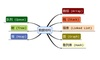数据结构 订阅